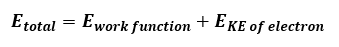# Problem: Find the velocity of an electron emitted by a metal whose threshold frequency is 2.25×1014 s-1 when it is exposed to visible light of wavelength 5.00×10−7 m.

###### FREE Expert Solution

Photoelectric effect occurs when photons (light) with enough energy hit the surface of a metal electrons are emitted. When dealing with photoelectric effect, we will use the following equation:Calculate Etotal from the light using the energy equation:###### Problem Details

Find the velocity of an electron emitted by a metal whose threshold frequency is 2.25×1014 s-1 when it is exposed to visible light of wavelength 5.00×10−7 m.